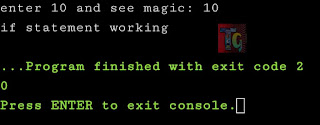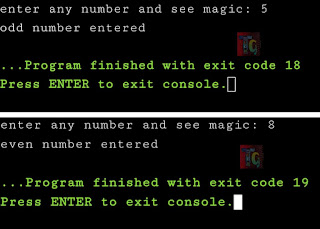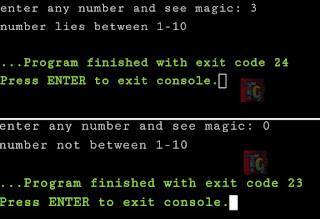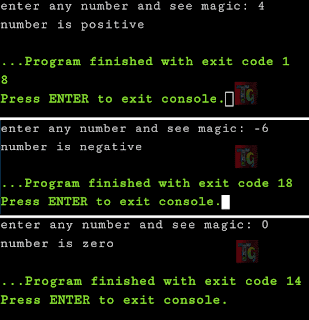# if Statement in C

Whenever in any C program you come across a decision-making statement if statement comes into play.if statement in C

## Nature of if statement in C

If statements in C are used to control the program flow based on preset condition. It is used to execute a single statement or a set of statements in a code block if the expression is evaluated to true. If false, it will get skipped.

## Types of if statement in C

Based on the situation and nature of a program, there are broadly four types of if statement in C program:

### Syntax of simple if statement

The following is basic structure of a simple if statement in C:

``````if(test_expression)
{
set of statements;
}``````

In the above code if the test_expression is satisfied only then the set of statements will be executed, else the code will be exited.

#### Flowchart of if statement:Flowchart of if statement

Sample program using if statement in C:

``````/*Use of if statement*/
#include<stdio.h>
void main()
{
int x=0;
printf("enter 10 and see magic: ");
scanf("%d",&x);
if(x=10)
printf("if statement working");
return 0;
}``````

#### Output:Output of if statement in C

### Syntax of if-else statement

The following is basic structure of an if-else statement in C:

``````if(test_expression)
{
set of statements;
}
else
{
set of alternate statements;
}
``````

In the above code if the test_expression is satisfied only then the set of statements from the if block will be executed, otherwise the code will be executed from else section.

#### Flowchart of if-else statement:Flowchart of if-else statement

Sample program using if-else statement in C:

``````/*Use of if-else statement*/
#include<stdio.h>
void main()
{
int x=0;
printf("enter any number and see magic: ");
scanf("%d",&x);
if(x%2==0)
printf("even number entered");
else
printf("odd number entered");
return 0;
}``````

#### Output:Two different results for different inputs

### Syntax of nested if-else statement

The following is basic structure of a nested if-else statement in C:

``````if(test_expression one)
{
if(test_expression two)
{
//When both test expression one and two holds true then the codes herein will be executed.
}
}
else
{
//set of statements in else block
}
``````

In the above code if the test_expression one is satisfied only then the checking of test_expression two will be held. If both holds true, then set of statements from the if block will be executed, otherwise the code will be executed from else section.

Sample program using nested if-else statement in C:

``````/*Use of nested if-else statement*/
#include<stdio.h>
void main()
{
int x=0;
printf("enter any number and see magic: ");
scanf("%d",&x);
if(x>1)
{
if(x<10)
printf("number lies between 1-10");
}
else
printf("number not between 1-10");
return 0;
}
``````

#### Output:Two Different results for different inputs

### Syntax of else-if statement

The following is basic structure of an else-if statement in C:

``````if(test_expression)
{
//set of statements
}
else if(test_expression n)
{
//set of other statements
}
else
{
//set of alternative statements
}
``````

In the above code if the test_expression is satisfied then block of codes under if statement will be executed. If that fails, then set of other statements from the else if block will be executed, otherwise the code will be executed from else section.

Sample program using else-if statement in C:

``````/*Use of else-if statement*/
#include<stdio.h>
void main()
{
int x=0;
printf("enter any number and see magic: ");
scanf("%d",&x);
if(x<0)
{
printf("number is negative");
}
else if(x>0)
printf("number is positive");
else
printf("number is zero");
return 0;
}
``````

#### Output:Three different results for different inputs

All programs written in this post are compiled online.

### Books I Prefer:I hope this article was helpful to understand the types and nature of if, nested if, else, else if statements in C! Comment below, if you've got any question. Head back soon for another interesting article on C Programming.